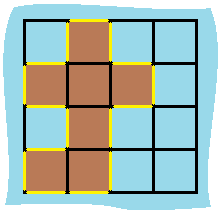Determine the perimeter of the island.
``package questionsimport _utils.UseCommentAsDocumentationimport utils.shouldBe/** * You are given row x col grid representing a map where grid[i][j] = 1 represents land and grid[i][j] = 0 represents water. * Grid cells are connected horizontally/vertically (not diagonally). * The grid is completely surrounded by water, and there is exactly one island (i.e., one or more connected land cells). * The island doesn't have "lakes", meaning the water inside isn't connected to the water around the island. * One cell is a square with side length 1. The grid is rectangular, width and height don't exceed 100. * * <img src="https://assets.leetcode.com/uploads/2018/10/12/island.png" height="150" width="150"/> * Determine the perimeter of the island. * * [Source](https://leetcode.com/problems/island-perimeter/) */@UseCommentAsDocumentationprivate fun islandPerimeter(grid: Array<IntArray>): Int {    var result = 0    val colSize = grid.lastIndex    for (row in 0..grid.lastIndex) {        for (col in 0..colSize) {            val current = grid[row][col]            if (current == 0) {                //  not a land                continue            }            val left = grid[row].getOrNull(col - 1)            val right = grid[row].getOrNull(col + 1)            val top = grid.getOrNull(row - 1)?.getOrNull(col)            val bottom = grid.getOrNull(row + 1)?.getOrNull(col)            // check if there is land on each of its direction            if (left == null || left == 0) result++            if (right == null || right == 0) result++            if (top == null || top == 0) result++            if (bottom == null || bottom == 0) result++        }    }    return result}fun main() {    islandPerimeter(        arrayOf(intArrayOf(0, 1, 0, 0), intArrayOf(1, 1, 1, 0), intArrayOf(0, 1, 0, 0), intArrayOf(1, 1, 0, 0))    ) shouldBe 16}``